Search

Solving Rational Inequalities (page 1 of 2)Solving rational inequalities is very similar to solving polynomial inequalities. But because rational expressions have denominators (and therefore may have places where they're not defined), you have to be a little more careful in finding your solutions.

To solve a rational inequality, you first find the zeroes (from the numerator) and the undefined points (from the denominator). You use these zeroes and undefined points to divide the number line into intervals. Then you find the sign of the rational on each interval.

• Solve the following:
•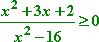First, I'll factor everything: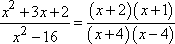This polynomial fraction will be zero wherever the numerator is zero, so I'll set the numerator equal to zero and solve:

(x + 2)(x + 1) = 0
x + 2 = 0  or  x + 1 = 0

x = 2  or  x = 1

The fraction will be undefined wherever the denominator is zero, so I'll set the denominator equal to zero and solve:

(x + 4)(x  4) = 0
x + 4 = 0  or  x  4 = 0

x = 4  or  x = 4

These four values, 4, 2, 1, and 4, divide the number line into five intervals, namely:

(infinity, 4), (4, 2), (2, 1), (1, 4), and (4, +infinity).

I could use "test points" to find the solution to the inequality, by picking an x-value in each interval, plugging it into the original rational expression, simplifying to get a numerical answer, and then checking the sign, but that process gets long and annoying (and is prone to errors), so I'll use the easier and faster factor-table method instead.

 My factor table looks like this:  My table has one row for each factor, a row for the number line, and a row for the rational expression. Each row is split into columns, with each column corresponding to one of the intervals on the number line.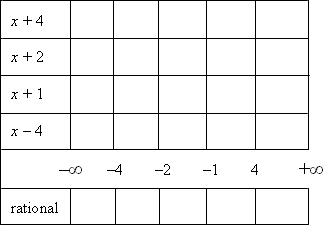The sign of the rational expression is a result of the signs of its various factors, so I need to find where each factor is positive:   Copyright © Elizabeth Stapel 2005-2011 All Rights Reserved

x + 4 > 0  for  x > 4
x + 2 > 0
for  x > 2
x + 1 > 0
for  x > 1
x  4 > 0
for  x > 4

Now I can put "plus" signs on the intervals in each row where that row's factor is positive: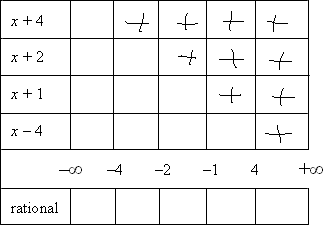Wherever a factor isn't positive, it's negative, so I'll put "minus" signs in the other intervals of each row: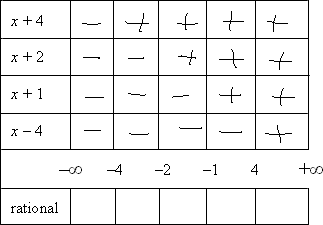Multiplying the signs down the columns, I get the overall sign on the original rational expression on each interval: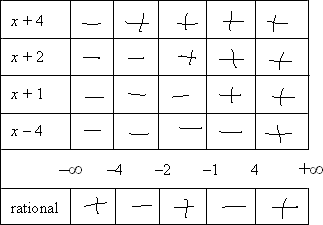Then the rational is positive on the intervals (infinity, 4), (2, 1), and (4, +infinity).

Looking back at the original exercise, this is an "or equal to" inequality, so I need to consider the interval endpoints, too. If this were a polynomial inequality, I could just throw all the interval endpoints into the solution, and I'd be done. For rationals, though, I have to be careful not to include any x-values that would cause division by zero.

The intervals' endpoints are 4, 2, 1, and 4. I can include 2 and 1 in the solution, because they just make the expression equal to zero by making the numerator zero. But plugging in 4 or 4 would cause division by zero, making the rational expression undefined, so I can't include these values in the solution.

Then the full solution is: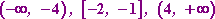If you have to write your solution in "inequality" notation, it would look like this:

x < 4, 2 < x < 1, and x > 4

Don't forget: "Infinity" is not a "number" in the way that, say, "2" is. "Infinity" cannot be "included" in your solution, so never draw a square bracket next to an "infinity" "endpoint".